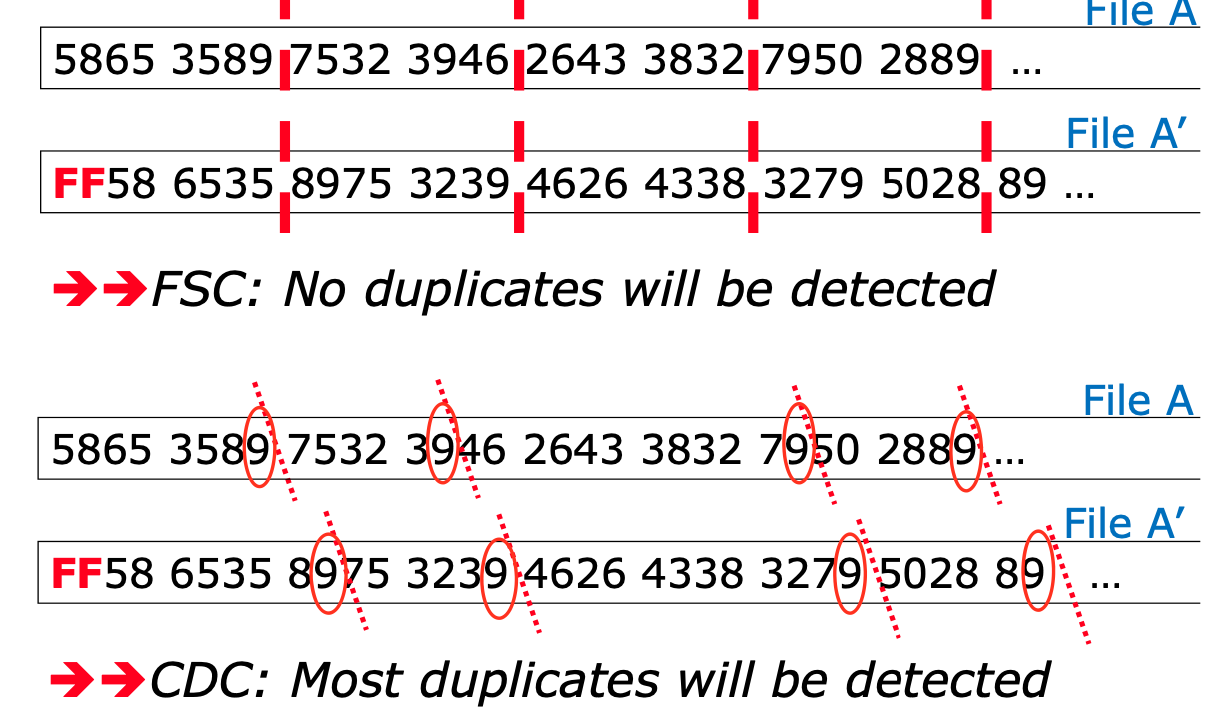# Content Defined Chunking

by Sevki
19 Jul 2022
[pdf and ps]

I'm not going to go in to much detail here, there are many excellent resources out there for this topic, but briefly content defined chunking is a technique that is used to break up a large file into smaller chunks.

The particular algorithm is called "FastCDC" as described in FastCDC: a Fast and Efficient Content-Defined Chunking Approach for Data Deduplication and it's corresponding presentation. You should go check these out.

FastCDC breaks up files in to chunk by using a rolling hash function to determine content boundaries.

This method compared to Fixed-Size Chunking is a bit more efficient, but it's also a catches more chunks than Fixed-Size Chunking.hopefully you'll have a better idea of what CDC does. Let's now see how that works in practice.

Let's build a simple deduplicator system The algorithm is as follows:

``````voters := map[string]chan chunk{}

totalbytes := map[string]int{}
popularBytes := map[string]int{}

for _, arg := range fs.Args() {
u, err := url.Parse(arg)
if err != nil {
fmt.Fprintf(os.Stderr, "error: %v\n", err)
os.Exit(1)
}
// check schemes, if empty use file
if u.Scheme == "" {
u.Scheme = "file"
}
voters[u.String()] = process(u, sha256.New(), *minSize, *maxSize, *bits)
totalbytes[u.String()] = 0
popularBytes[u.String()] = 0
}

election := combine(voters)

epoch := 0
for round := range election {
epoch++
// print the epoch in magenta
fmt.Printf("\x1b[35m%d\x1b[0m\n", epoch)
// every round find the most popular chunk
// by going through all the votes and finding the most popular score
tally := map[uint64]int{}
for _, chunk := range round {
tally[chunk.score]++
}

// find the most popular score
var maxScore uint64
for score, count := range tally {
// if the count is greater than the current max and is more than 1
if count > 1 && count > tally[maxScore] {
maxScore = score
}
}
// if the most maxScore is 0, then we have don't have a winner
if maxScore == 0 {
continue
}
// print the most popular score in green
fmt.Printf("\x1b[32m%b\x1b[0m\n", maxScore)
// print the chunks with the most popular score in green and the rest in red

for filename, chunk := range round {
if chunk.score == maxScore {
// print it in green
fmt.Printf("\x1b[32m%s: %s\x1b[0m", filename, chunk.String())
popularBytes[filename] += len(chunk.data)
} else {
// print it in red
fmt.Printf("\x1b[31m%s: %s\x1b[0m", filename, chunk.String())
}
totalbytes[filename] += len(chunk.data)
}
}
// for each file, print the total bytes and the popular bytes and it's percentage
for filename, total := range totalbytes {
fmt.Printf("%s: %d (%d%%)\n", filename, total, popularBytes[filename]*100/total)
// print the popular bytes in green
}
``````

After we determine if a chunk is popular, we can use this to determine if a chunk is a duplicate.

For instance the following go programs

``````// 1.go
package main

import "fmt"

func main() {
fmt.Println("Hello, 世界")
}
``````
``````// 2.go
package main

import "fmt"

func main() {
fmt.Println("Hello, World!")
}
``````
``````// 3.go
package main

import "fmt"

func main() {
fmt.Println("Hello, World!")
}
``````
``````go build -o testdata/1.out -trimpath -ldflags=-buildid= testdata/1.go
go build -o testdata/2.out -trimpath -ldflags=-buildid= testdata/2.go
go build -o testdata/3.out -trimpath -ldflags=-buildid= testdata/3.go

go run ../../cmd/dedupe *.out | aha > out.html
``````

Since go programs are reproducible, we'd expect `2.out` and `3.out` to be duplicates and would expect `1.out` to be be sharing most of the code.

And indeed the results does confirm the assumption

``````file://1.out: 1826997 (72%)
file://2.out: 1844660 (100%)
file://3.out: 1844660 (100%)
``````

check out the result

This is a very simple example, but it's a good starting point for deduplication.

Next steps should be to find something like a docker registry and see how much data that can be deduplicated there.